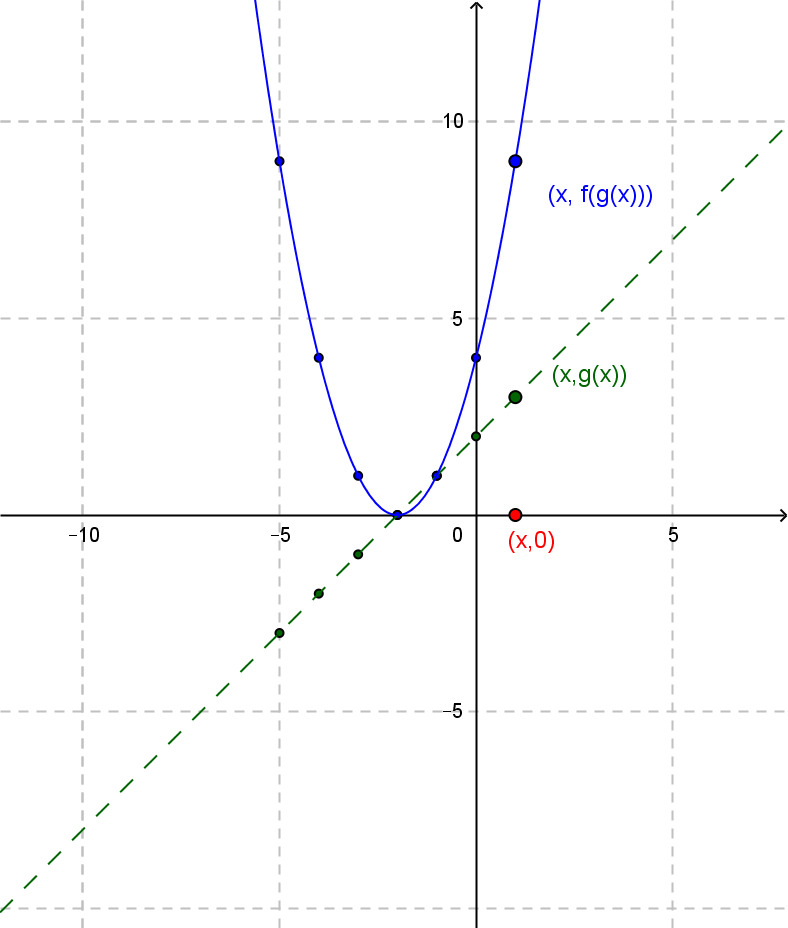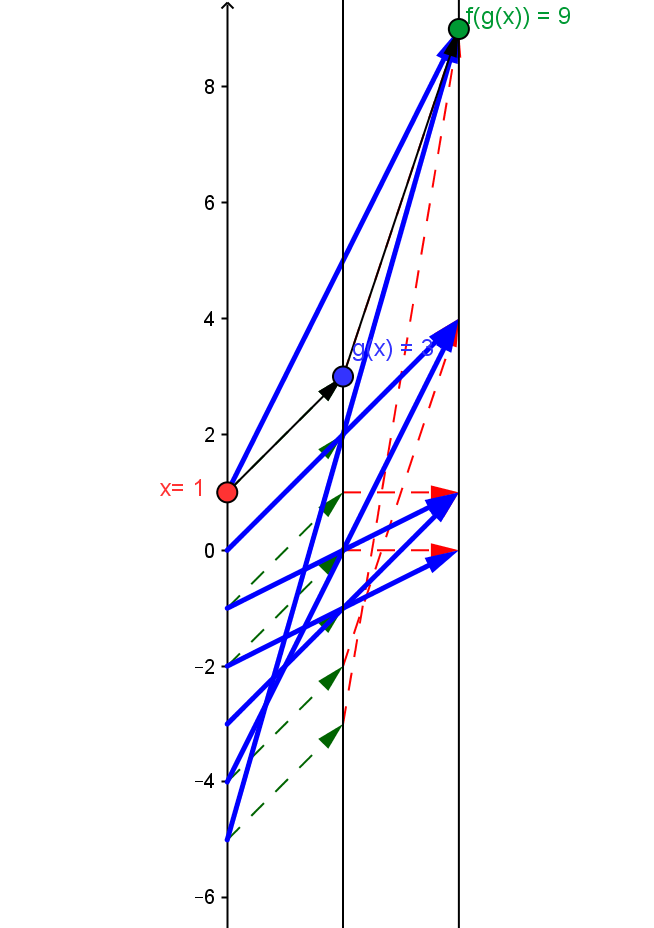Example QF.3.2 : \$h =-2; q(x) = (x+2)^2\$
This is best understood as the composition of the linear function \$g(x)= x+2\$ followed by the core quadratic function \$f(x) = x^2\$, so \$q(x) = f(g(x))\$.
Draw a mapping diagram yourself or  use the diagram created with GeoGebra to explore the diagram further.
Compare the mapping diagram with the graphs of \$g(x)\$ and \$f(x)\$
 Graphs of \$g(x)\$ and \$f(x)\$Mapping Diagram Showing Composition. Given a point / number, \$x\$, on the source line, there is a blue arrow  meeting the target line at the point / number, \$(x+2)^2\$ This point corresponds to the quadratic function's value for \$x\$. The values for the core mapping diagram for \$x^2\$ in redare  applied to the values \$g(x)=x+2\$ (green).

As \$x\$ increases, \$q(x)\$ decreases to value \$q(-2)=0\$ and then increases.